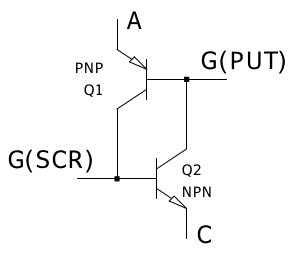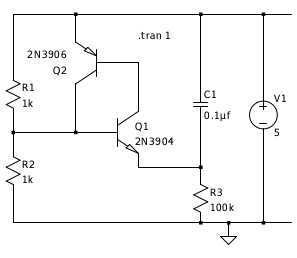# PUT part three

started 15th March 2020

SCR Oscillator

A Programmable Unijunction Transistor (PUT) is a four layer pnpn device as is a thyristor aka Silicon Controlled Rectifier (SCR). The difference is which layer is connected to the gate - for a PUT p(A)n(G)pn(C) for an SCR p(A)np(G)n(C) - where A is the anode, C cathode and G the gate. Looking at the transistor equivalent circuit.From the circuit for the PUT oscillator:symmetry gives an oscillator circuit for the SCR:Or with a real SCR:(a 2N6027 is a PUT, however the ones I got from ebay were SCRs and work in this circuit).

PUT Pulse stretcher

Imagine you have a vibration switch that outputs pulses and a micro-controller that can't see them because they are too fast. The micro-controller interface is a single pin which has a pull up resistor giving 5 V through a 10 K resistor, this is the only power available. To signal to the micro-controller the pin has to be pulled close to 0 V.

This circuit does the trick. In the 'scope traces, yellow is the switch signal and red the voltage across the circuit equal to the signal on the micro-controller input pin.

It is possible to replace the diode with a 100 K resistor, the pulse is then a bit shorter. In this diagram the 2N6027 is an actual real 2N6027 PUT, despite the symbol being that for an SCR.

You could look at this circuit as using a monostable to debounce a switch. However the novelty is having no independent power supply, just the power that is used to sense the switch state - I am sat outside a black box and I choose not to enter it or have an external power source.